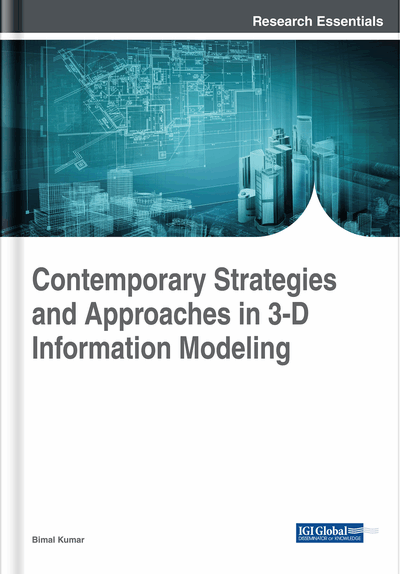# Topologically Consistent Space, Time, Version, and Scale Using Alexandrov Topologies

Patrick E. Bradley (Karlsruhe Institute of Technology, Germany) and Norbert Paul (IEP Industrie Elektronik Paul GmbH, Germany)
DOI: 10.4018/978-1-5225-5625-1.ch003
Available
\$37.50
No Current Special Offers

## Abstract

A novel approach to higher dimensional spatial database design is introduced by replacing the canonical solid–face–edge–vertex schema of topological data by a common type SpatialEntity, and the individual “bounded-by” relations between two consecutive classes by one separate binary relation BoundedBy on SpatialEntity defining an Alexandrov topology. This exposes mathematical principles of spatial data design. The first consequence is a mathematical definition of topological “dimension” for spatial data. Another is that every topology for spatial data is an Alexandrov topology. Also, version histories have a canonical Alexandrov topology, and generalizations can be consistently modeled by continuous foreign keys between LoDs. The result is a relational database schema for spatial data of dimension 6 and more, seamlessly integrating space-time, LoDs, and version history. Topological constructions enable queries across these different aspects. Giving points coordinates amounts can give rise to topological inconsistencies which can be measured with topological invariants.
Chapter Preview
Top

## Introduction

2D and 3D spatial models are well established for spatial data modeling, and there exist standards like CityGML in geo-spatial modeling and IFC for architectural models. These standards cover the representation of geometric objects – mostly using an incidence-based representation like, for example, (Rossignac & O’Connor, 1989; Hazelton et al., 1990). Currently there is active research on spatio-temporal queries (Sakr & Güting, 2009) as well as 4D spatio-temporal modeling (Anh, Vinh, & Duy, 2012), and also on considering other aspects like scale (v. Oosterom & Stoter, 2012) as additional dimensions of spatial data. Besides that, research on nD modeling provides generic spatial data models without a fixed dimension upper bound (Lienhardt, 1994) and often gives a formal definition of “topological dimension” of spatial data. Lienhardt proposes an nD data model called G-Maps where spatial entities and their topology are only implicitly given. Both (Bradley & Paul, 2014) and (Lienhardt, 1991) contain a broad discussion of the differences between G-Maps and incidence-based models as the one presented in this article. Topology has its own sub-discipline called “dimension theory” (Engelking, 1978) where the possible definitions of “topological dimension” are investigated. Among these, the Krull dimension (Hartshorne, 1993, p. 5) is particularly applicable for spatial data and is proposed in this article as a standard definition of spatial data dimension.

As a result of investigations if data of dimension beyond 4 have practical applications and if this could possibly lead to a “combinatorial explosion of complexity”, and inspired by van Oosterom’s ideas (v. Oosterom & Meijers, 2011), this article demonstrates the mathematical foundations of generic nD spatial modeling and its use for combining 3D spatial data, time, scale, and versioning into an integrated 6D+ model. In particular, it will be shown that sensible integration of scale increases the dimension of spatial data by more than one, hence the “+” in 6D+. Even more, it has turned out that further increasing the dimension can even decrease the complexity of the data model.

First a short introduction into the basic concepts of mathematical topology is given. This also covers a discussion of incidence graphs which turn out to be topological spaces. Then, as a small example application, an SQL representation of Egenhofer’s (1991) nine-intersections model is presented. Surprisingly, there are SQL queries that prove the yet unverified mathematical statements in (Egenhofer, 1991) wrong. As the main intent is to expose mathematical principles of nD modeling, the relational model, formulated by Codd (1990), is used because with set theory it has the same mathematical foundation as topology (Bradley & Paul, 2010). The principles described here are also applicable to object oriented modeling. They also serve as a basis for a generic topological database model as an extension of the relational model: instead of tables, there are spaces on which queries operate, and then they return spaces as a result. Then, the relational database schema of the 6D+ model is developed step by step. By assigning coordinates to points, topological inconsistencies can occur. The concept of topological consistency is defined rigourously, and it is explained how to measure topological inconsistency. Finally, an implementation of concepts from this article is discussed.

## Complete Chapter List

Search this Book:
Reset